# FLARE On Challenge (2015) #6

This challenge is an Android application. There are a lot of tools can be used to analyze Android application and the JEB Decompiler is my favorite one. Let’s open this challenge in JEB and look at the Manifest file at first: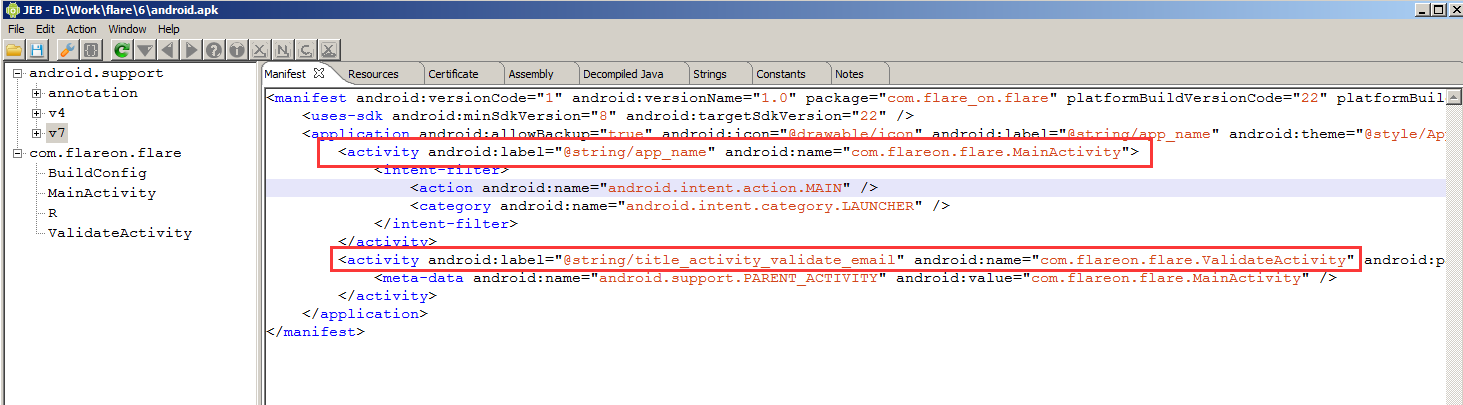The Manifest gives us many information and the most important are the two Activity: com.flareon.flare.MainActivity and com.flareon.flare.ValidateActivity.

Let’s see what will the MainActivity do: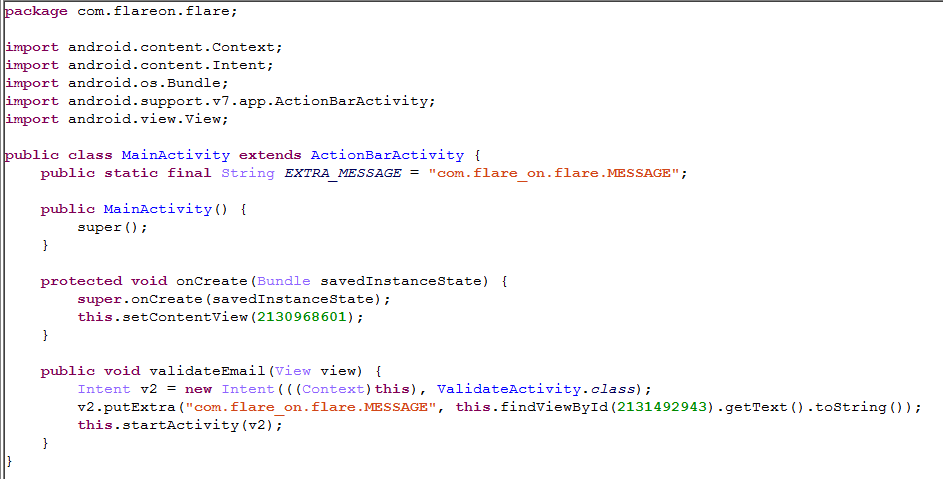The functionality looks simple: it reads the input and stores it as an extra data named com.flare_on.flare.MESSAGE, and then it launches the ValidateActivity. So we move to the ValidateActivity: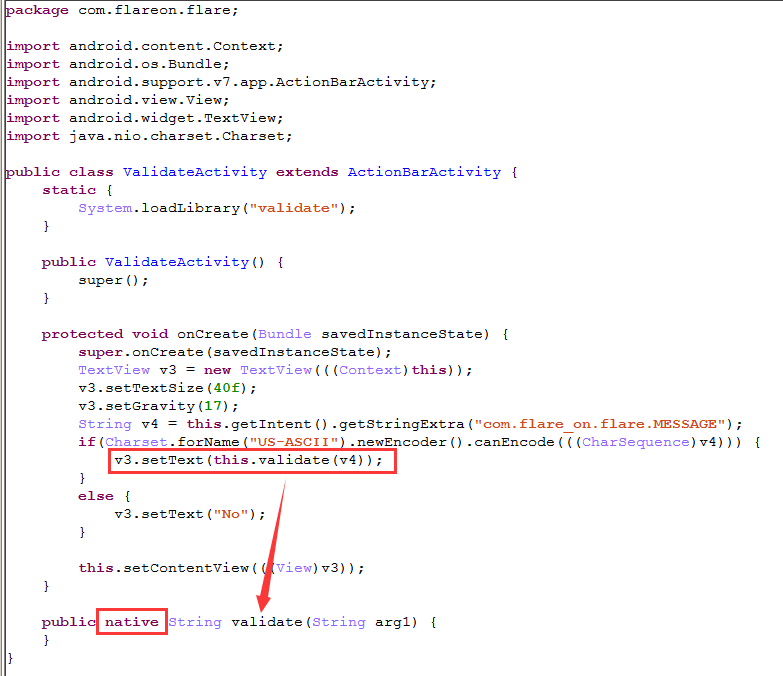The ValidateActivity will retrieve the extra data stored in the com.flare_on.flare.MESSAGE, then verify if it is an ASCII string, if so it will pass this string to a function named “validate”, if not it will set the text “No”. So what does the function validate() do? From the keyword “native” in the function definition we can know that this is actually a native function which could exist in a native library. If you decompress the APK file with a decompression tool like 7-zip you may notice that there is a folder named lib and inside this folder there is an interesting file named libvalidate.so, from the file name we can guess that this one should be the file we want. So let’s disassemble it in IDA. From the Entry Point List (Ctrl + E) we can see a function named Java_com_flareon_flare_ValidateActivity_validate, which is interesting: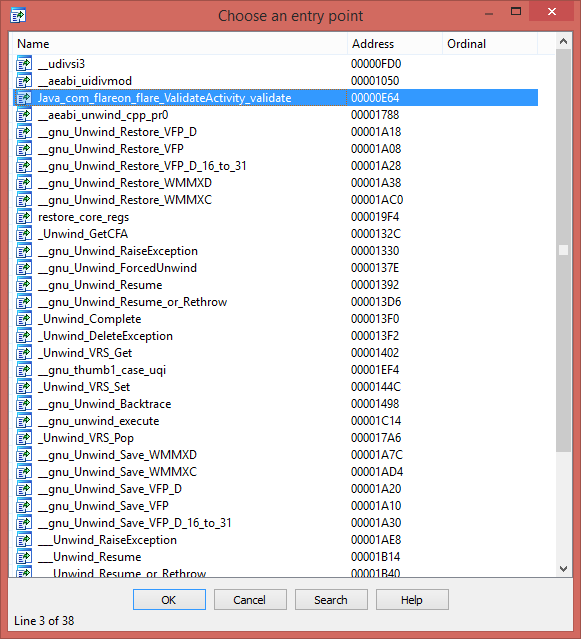A quick navigate on this function we can find that there are two strings “That’s it!” and “No” present at the end of this function: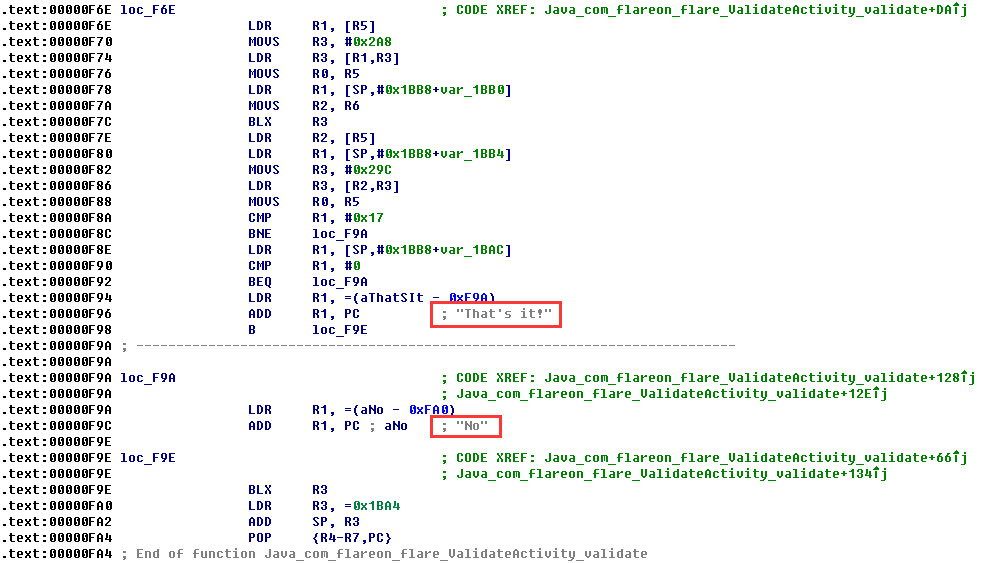Those strings confirm that this function is what we need, so the next thing to do is no more than read the disassembly code to understand the functionality of this function. In short, this function will verify if the length of the input string is equal to or less than 46 at first, then it will convert two adjacent bytes of the input string to a 16-bit WORD (e.g. if two adjacent bytes are 0x11 and 0x22, they will be converted to 0x1122), and next, it will calculate the remainder of the WORD by dividing each 16-bit WORD in a table (I call it mod_table) located at address 0x00002214, if the remainder is zero, it will increase the relevant byte in another table (I call it reminder_table) by 1, and use the dividing result as a new DWORD to calculate the remainder until the dividing result equal to or less than 1. And finally it will compare the reminder_table with a hard-coded table (I call it key_table) to see if they are match. The following pseudo code may help you understand the flow:

```if (input.length > 46)
return "No"

result = 0
for (i = 0, table_index = 0; i <= input.length; i += 2, table_index++)
{
n = (input[i] << 8) | input[i+1]

j = 0
while (j < 0xD94)
{
while ((n % mod_table[j]) == 0)
{
reminder_table[table_index][j] += 1
n = n / mod_table[j]
if (n <= 1)
goto label_next
}
j++
}
label_next:
if (reminder_table[table_index] == key_table[table_index])
{
result += 1
}
}

if (result == 0x17)
return "That's it!"

return "No"
```

Obviously, this algorithm can be easily reversed, the following IDA Python script can be used to calculate the correct input:

```from idaapi import *
import struct

mod_table = 0x00002214
key_tables = 0x00005004
key_tables_len = 0x17
key_table_len = 0xD94
out_str = ''

for i in range(0, key_tables_len):
key_table = Dword(key_tables + i*4)
out = 1
for j in range(0, key_table_len):
index = Word(key_table + j*2)
if index != 0:
num = Word(mod_table + j*2)
out = out * (num ** index)
out_str += struct.pack('<H', out)[::-1]

print out_str
```
This entry was posted in CTF and tagged , , , . Bookmark the permalink.